Your browser does not support the IFRAME tag.Home MonkeyNotes Printable Notes Digital Library Study Guides Study Smart Parents Tips College Planning Test Prep Fun Zone Help / FAQ How to Cite New Title Request

 CHAPTER 8 : APPLICATIONS OF THE DEFINITE INTEGRALS 8.1 Introduction In this chapter we shall learn how to apply the technique of the Definite Integral to obtain areas, volumes, mean values, work done, fluid pressure etc. For the evaluation of areas and volumes it is necessary to know the nature of the curve. Hence we shall study below the shapes of certain well-known curves. (1) Straight Line :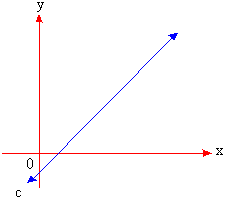The general for + m : ax + by c = 0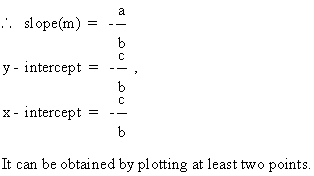(2) Circle :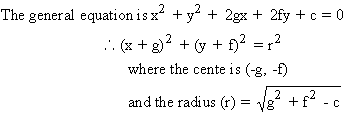(3) (a) Standard Parabola : The general equations of the parabolas are Y2 = 4 ax,   y2 = - 4 ax,   x2 = 4 by    and    x2 = -4 by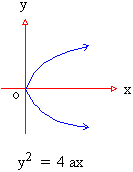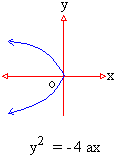Index 8.1 Introduction8.2 Area 8.3 Volumes 8.4 Mean Value 8.5 Arc Length(Rectification) Chapter 1